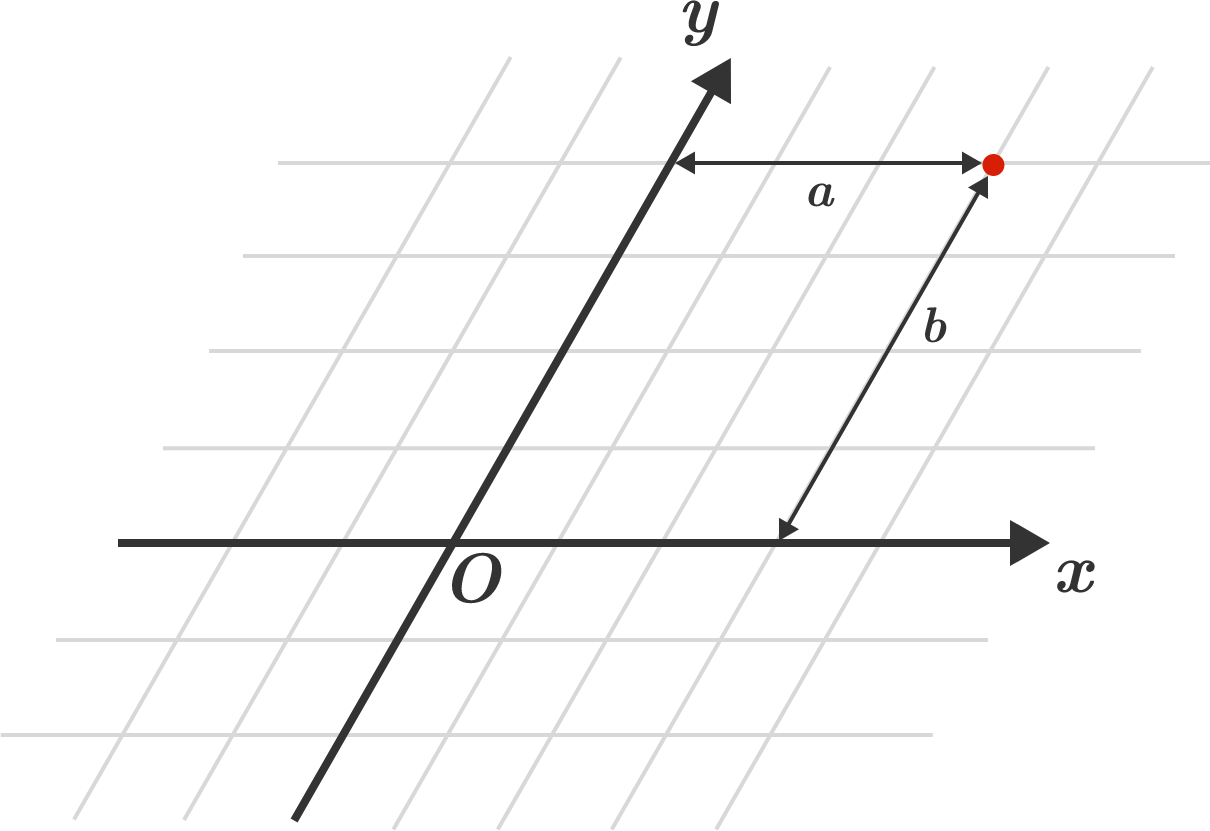# Oblique Coordinates

Geometry Level 1Did you know that we can have coordinate systems where the coordinate axes are not perpendicular to each other? Such coordinate systems are known as oblique coordinate systems.

The above figure shows an oblique coordinate system. In such systems, the $x$-coordinate of a point is found by measuring the distance from the point to the $y$-axis parallel to the $x$-axis. Similarly, the $y$-coordinate of a point is found by measuring the distance from the point to the $x$-axis parallel to the $y$-axis. The red point in the above figure has the coordinates $(a, b)$.

Question: We are given two points: $A \ (1,6)$ and $B \ (5,2)$ in an oblique coordinate system where the angle between the positive axes is $60^{\circ}$. What is the distance between the two points, $AB$?

×

Problem Loading...

Note Loading...

Set Loading...